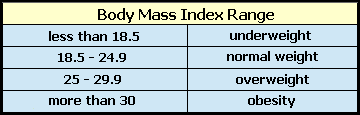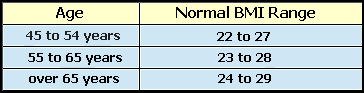Spike's Calculators

# BMI Calculator Using Stones and Pounds

Calculate a person's body mass index (BMI) number using feet and inches for length, stones and pounds for body weight.```
Note:The body mass index range does not take into account whether the weight is carried as muscle or fat!```

The BMI range is adjusted with age. For normal weight:### BMI From Stone and Pound

Your Weight in Stones and Pounds st lbs
Your Height in Feet ft in n
d

 BMI #

#### Calculation

1. enter the weight in stones and pounds
2. the length in feet and inches

#### Results

1. BMI number

##### Formula
```BMI = weight in pounds %divide; (height in inches)² * 703
BMI = body mass index
703 a constant used to convert pound/inch to kilogram/metre
```
```one pound (lb avdp) = 0.45359237 kilograms (kg)
one inch (in)= 0.0254 metres (m)
0.45359237 %divide; 0.0254² = 703.0695796391592783 rounded to 703
```
/div>

### BMI Calculators

Calculate your body mass index (BMI) using different combinations of units to measure humans' weight and height.